# Some applications of algebraic number theory

The following examples are meant to convince you that learning algebraic number theory now will be an excellent investment of your time. If an example below seems vague to you, it is safe to ignore it.

1. Integer factorization using the number field sieve. The number field sieve is the asymptotically fastest known algorithm for factoring general large integers (that don't have too special of a form). Recently, in December 2003, the number field sieve was used to factor the RSA-576 \$10000 challenge: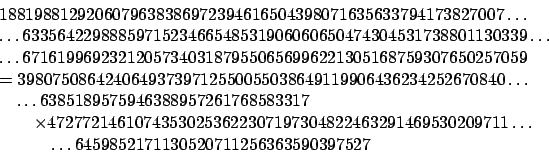(The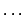indicates that the newline should be removed, not that there are missing digits.) For more information on the NFS, see the paper by Lenstra et al. on the Math 129 web page.
2. Primality test: Agrawal and his students Saxena and Kayal from India recently (2002) found the first ever deterministic polynomial-time (in the number of digits) primality test. There methods involve arithmetic in quotients of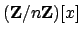, which are best understood in the context of algebraic number theory. For example, Lenstra, Bernstein, and others have done that and improved the algorithm significantly.

3. Deeper point of view on questions in number theory:
1. Pell's Equation (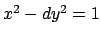)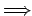Units in real quadratic fieldsUnit groups in number fields
2. Diophantine EquationsFor which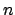does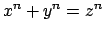have a nontrivial solution in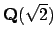?
3. Integer FactorizationFactorization of ideals
4. Riemann HypothesisGeneralized Riemann Hypothesis
5. Deeper proof of Gauss's quadratic reciprocity law in terms of arithmetic of cyclotomic fields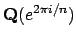, which leads to class field theory.
4. Wiles's proof of Fermat's Last Theorem, i.e.,has no nontrivial integer solutions, uses methods from algebraic number theory extensively (in addition to many other deep techniques). Attempts to prove Fermat's Last Theorem long ago were hugely influential in the development of algebraic number theory (by Dedekind, Kummer, Kronecker, et al.).
5. Arithmetic geometry: This is a huge field that studies solutions to polynomial equations that lie in arithmetically interesting rings, such as the integers or number fields. A famous major triumph of arithmetic geometry is Faltings's proof of Mordell's Conjecture.

Theorem 2.3.1 (Faltings)   Let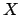be a plane algebraic curve over a number field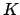. Assume that the manifold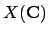of complex solutions tohas genus at least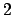(i.e.,is topologically a donut with two holes). Then the set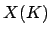of points onwith coordinates inis finite.

For example, Theorem 2.3.1 implies that for any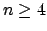and any number field, there are only finitely many solutions into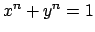. A famous open problem in arithmetic geometry is the Birch and Swinnerton-Dyer conjecture, which gives a deep conjectural criterion for exactly whenshould be infinite whenis a torus.

William Stein 2004-05-06